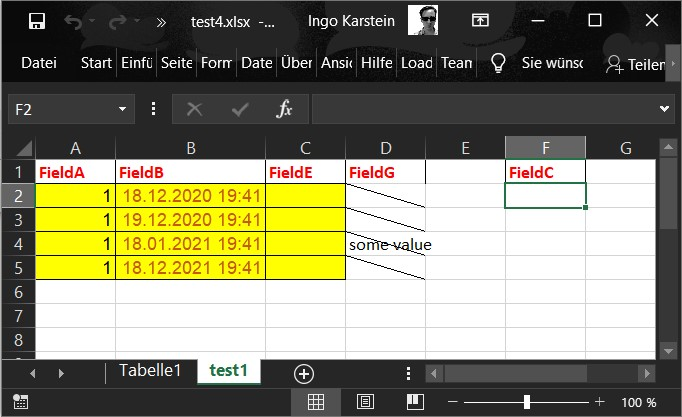# New-KFExcelFile

## Syntax

``````New-KFExcelFile
-Input <list-of-hashtables>
-SheetName <string>
-AsStream
[-InFile <template-excel-file>]
[-Fields <list-of-strings>]
[-ThrowErrors[:\$true]]

New-KFExcelFile
-Input <list-of-hashtables>
-SheetName <string>
-AsBase64
[-InFile <template-excel-file>]
[-Fields <list-of-strings>]
[-ThrowErrors[:\$true]]

New-KFExcelFile
-Input <list-of-hashtables>
-SheetName <string>
-OutFile <path>
[-InFile <template-excel-file>]
[-Fields <list-of-strings>]
[-ThrowErrors[:\$true]]
``````

## Support

>= kenaflow 4.0.10

## Return

In case of using `-AsStream` it returns a `System.IO.MemoryStreaam` object.

In case of using `-AsBase64` it returns a string.

In case of using `-OutFile` it returns nothing.

## Description

This cmdlet can be used to convert a list of PowerShell hashtables to an MS Excel file.

This is a such a list of hashtables:

``````@(
@{FieldA = "kenaflow"; FieldB = 1; FieldC = [DateTime]::Now}
@{FieldA = "is" ; FieldB = 2                               ; FieldD = 10.101}
@{FieldA = "cool"; FieldB = 3;
)
``````

There are three different output options:

1. as System.IO.MemoryString by using `-AsStream`
2. as Base64 encoded byte array using `-AsBase64`
3. or as file on disk using `-OutFile <path>`

You can only use one output option.

You have to specify the input object with `-Input` and the name of the workbook sheet using `-SheetName`.

If not using `-Infile` the cmdlet creates a new file as output.

If you specify an existing Excel file with `-InFile <template-excel-file>` you have to 3 options:

1. Specify the input file content as Base64 encoded string or
2. specify the input file as System.IO.Sream object or
3. speficy a full or relative path to an existing file on disk. It's relative to the current workflow folder.

With the input file you can do two things:

1. If the worksheet named with `-SheetName` already exists the cmdlet will take the field names from the 1st row. Than it will take the formatting for the data rows from the 2nd row. Everything starting with row 2 will be removed from the excel file. But no other worksheet is modified! The cell formats are not modified.

2. If the worksheet named with `-SheetName` does not exist it will be added using the default styling.

## Parameters

`-Input <list-of-hashtables>`

The inpupt object: a List of PowerShell hashtables

`-SheetName <string>`

The name of the Excel worksheet.

`-AsStream`

The result is a System.IO.MemoryStream object

`-AsBase64`

The result is a Base64 encoded byte array that represents the Excel file.

`-OutFile <path>`

The result is written to a file on disk. The path can be relative to the current workflow folder.

`[-InFile <template-excel-file>]`

An Excel file as template. The new worksheet will be added or the existing worksheet will be modified.

1. The template can be specified as Base64 encoded string or
2. as System.IO.Sream object or
3. as a full or relative path to an existing file on disk. It's relative to the current workflow folder.

`[-Fields <list-of-strings>]`

The field order can be specified here. If used only the specified fields are used as keys and read from the hastable objects in the list specified with `-Input`.

`[-ThrowErrors[:\$true]]`

This is a default parameter for all kenaflow cmdlets. If set to `\$true` (default!) the engine will pass exception within the cmdlet to the script for further handling.

## Example

``````New-KFExcelFile -input @(
@{FieldA = "kenaflow"; FieldB = [DateTime]::Now; FieldC = 10.101}
@{FieldA = "is"; FieldB = [DateTime]::Now.AddDays(1); FieldC = 10.101}
@{FieldA = "cool"; FieldB = [DateTime]::Now.addmonths(1); FieldC = 10.101}
@{FieldA = "!"; FieldB = [DateTime]::Now.addyears(1); FieldC = 10.101}
) -OutFile "test1.xlsx"  -SheetName "test1"
``````

Result: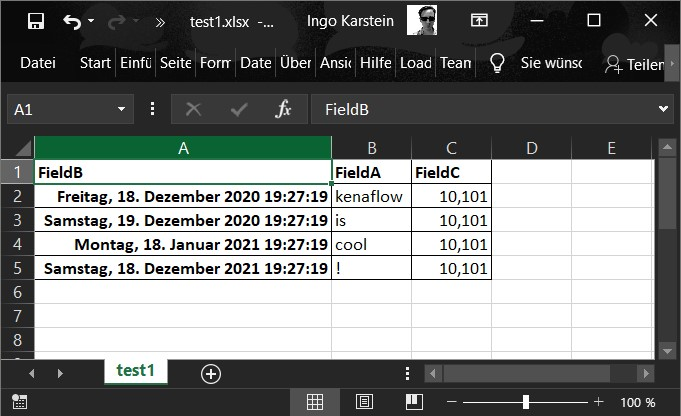``````New-KFExcelFile -input @(
@{FieldA = 1; FieldB = [DateTime]::Now; FieldC = 10.101; FieldD = "Hi"}
@{FieldA = 1; FieldB = [DateTime]::Now.AddDays(1); FieldC = 10.101; FieldD = "Hey"}
@{FieldA = 1; FieldB = [DateTime]::Now.AddMonths(1); FieldC = 10.101; FieldD = "Salut"}
@{FieldA = 1; FieldB = [DateTime]::Now; FieldC = 10.101; FieldD = "Hallo"}
) -Fields @("FieldA", "FieldB", "FieldD") -OutFile "test2.xlsx" -SheetName "Test2"
``````

Result: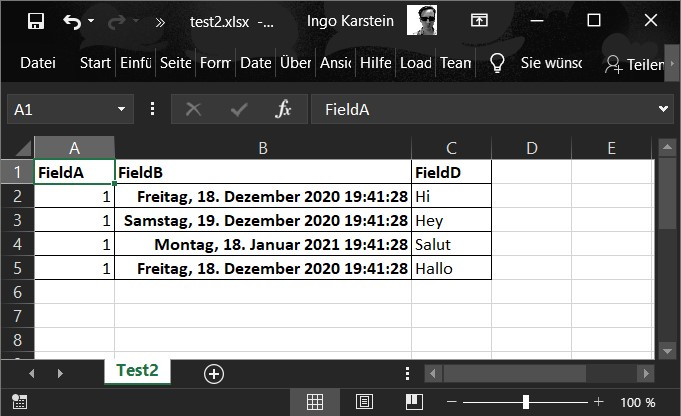``````New-KFExcelFile -input @(
@{FieldA = 1; FieldB = [DateTime]::Now; FieldC = 10.101; FieldD = "Hi"}
@{FieldA = 1; FieldB = [DateTime]::Now.AddDays(1); FieldC = 10.101; FieldD = "Hey"}
@{FieldA = 1; FieldB = [DateTime]::Now.AddMonths(1); FieldC = 10.101; FieldD = "Salut"}
@{FieldA = 1; FieldB = [DateTime]::Now.AddYears(1); FieldC = 10.101; FieldD = "Hallo"}
) -Fields @("FieldA", "FieldB", "FieldE") -OutFile "test3.xlsx" -InFile "\$PsscriptRoot\inp.xlsx" -SheetName "test1"
``````

Template file: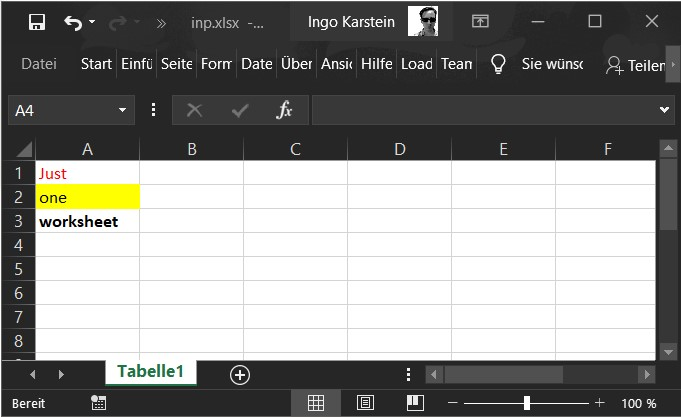Result: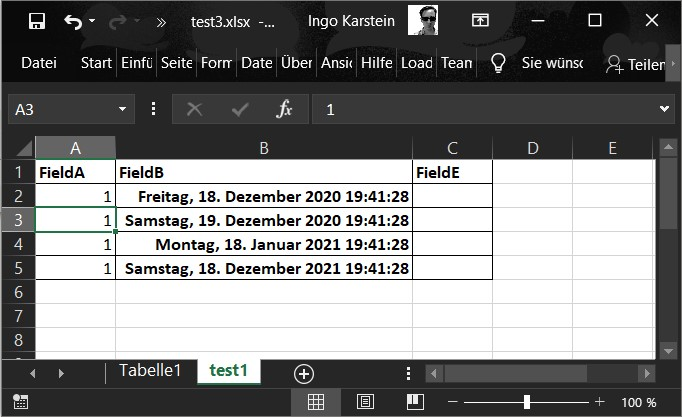``````New-KFExcelFile -input @(
@{FieldA = 1; FieldB = [DateTime]::Now; FieldC = 10.101; FieldD = "Hi"}
@{FieldA = 1; FieldB = [DateTime]::Now.AddDays(1); FieldC = 10.101; FieldD = "Hey"}
@{FieldA = 1; FieldB = [DateTime]::Now.AddMonths(1); FieldC = 10.101; FieldD = "Salut"; FieldG="some value"}
@{FieldA = 1; FieldB = [DateTime]::Now.AddYears(1); FieldC = 10.101; FieldD = "Hallo"}
) -OutFile "test4.xlsx" -InFile "\$PsscriptRoot\inp2.xlsx" -SheetName "test1"
``````

Template File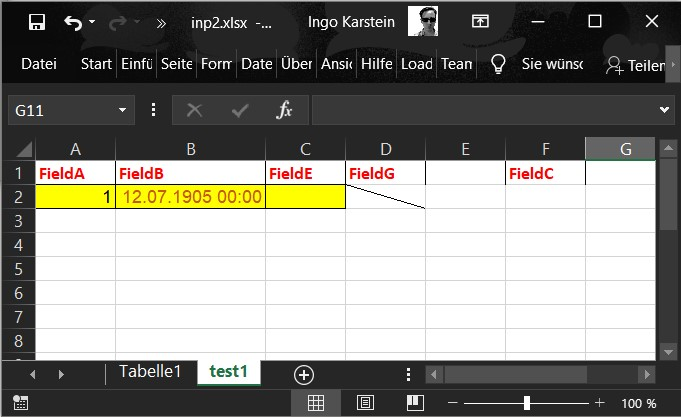Result